# Assignments

If you want to solve trigonometric equations it is necessary that you know quite a few trigonometric formulas, see also Trigonometry (Unit circle and simple formulas) and Trigonometry (Addition and subtraction formulas). It is also important that you know how to use the unit circle.

1. Solve: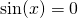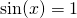Solution

2. Solve: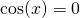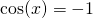Solution

3. Solve the following equations: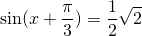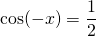Solution

4. Solve the following equation: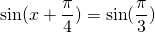Solution

5. Solve the following equation: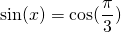Solution

6. Solve the following equation for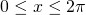.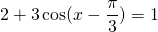Solution

7. Solve the following equation for: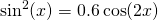Solution

8. Solve the following equation for: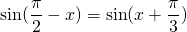Solution

9. Solve the following equation for: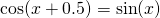Solution

10. Solve the following equation for: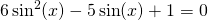Solution

0